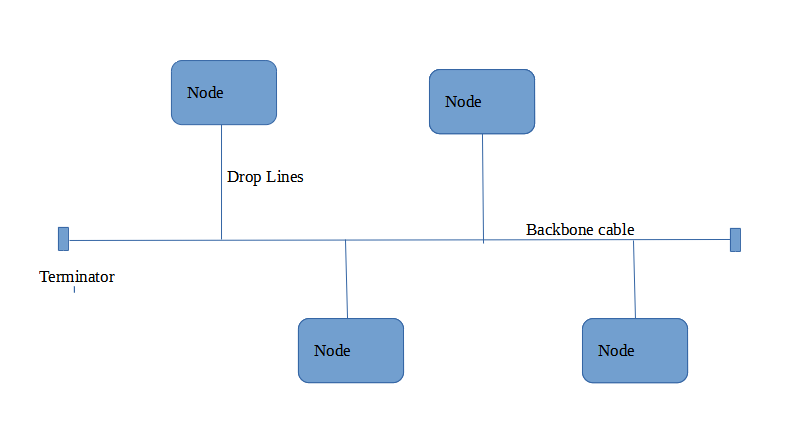# What do you mean by network topology? Explain Types

### Abstract

• Network topology is the arrangement of nodes and links of a network.
• Topologies are categorized as either physical network topology or logical network topology.
• The topology of a network is key to determining its performance.
• Network topology can be categorized into – Bus Topology, Ring Topology, Star Topology, Mesh Topology, and Tree Topology.

### Scope of Article

The article gives a basic understanding of what is network topology and what are various types of network topologies and how different topologies affect the performance of the network.

### Definition:

Network topology tells us about the way the links and nodes/devices are connected i.e., it refers to the layout or arrangement of nodes and links in a computer network.

### Categories of network topology:

• Physical Topology: Describes the placement of various nodes in a computer network. In other words, it shows how network devices are physically connected.
• Logical Topology: Describes the idea of in what way different nodes are connected and how the data is transmitted through the network. So logical topology deals with the data flow in the network.

Now let us understand why we need network topology.

Network topology plays a key role while establishing a network, some reasons are:

• Based on the topology used, network performance will be impacted.
• Topology acts as a factor in determining the media type used to cable the network.
• Depending on the topology cost of the network will be affected.

### Types of Network Topologies:

• Bus Topology:

It is a multipoint connection in which every node/device is connected to a single cable (Backbone cable) via drop lines. Bus topology consists of two ends and data is transmitted from one end to another in a single direction i.e., bus topology is unidirectional. Terminators are used at the end of the cable so that the electrical signal does not bounce back.If the bus topology contains N nodes, then the number of cables required to connect them will be equal to N+1 where N is the number of drop lines and 1 for the backbone cable.

• Requires a lesser number of cables.
• Easy to install and extend.
• Suitable for temporary and small networks.

• Fault detection is difficult.
• The entire network shuts down if the main cable breaks.
• Limited cable length.
• Transmission speed decreases when more devices are added to the network.
• Low security.
• Ring Topology:

In a ring topology, all the nodes are connected to form a ring such that every node will have exactly two neighbors.

The signal is passed in one direction from one device to another device until it reaches its destination. For the signal to reach from one point to another it must travel through all the intermediate nodes.

If the ring topology contains N nodes, then the number of cables required to connect them will be equal to N.

• Point-to-point connectivity of the nodes makes it easy to detect and identify faults.
• Easy to install.
• Cost-effective and inexpensive to install.
• Extending or reducing a node from topology is easy as only two links are required to be changed.
• The risk of collision is less as only one node is allowed to send data at a time.

• If a single node or a transmission line fails, the entire network breaks down.
• Communication delay increases as the number of nodes increases.
• Less secure.
• Star Topology:

In star topology, every device in the network is connected to a central device called a hub.

All communications are done through the hub i.e. if one device wants to send data to another device, it first has to send data to the hub, and then the hub sends the data to the designated target.

If the star topology contains N nodes, then the number of cables required to connect them will be equal to N.

• Easy to install and modify.
• Failure of one node will not affect the entire network.
• Easy to detect the failure.
• Less cabling is needed.

• If the central hub breaks down, the whole network collapses.
• Too much dependency on the central device.
• Mesh Topology:

In a mesh topology, each device is connected to every other device on the network through a dedicated point-to-point link. So, if there are N nodes in the network then every single node is connected to the N-1 number of nodes.

If the mesh topology contains N nodes, then the number of cables required to connect them will be equal to N*(N-1)/2.

• No data traffic issues because of the dedicated link between two devices.
• Failure of a link or a device doesn’t affect the entire network.
• Because of the dedicated point-to-point link, this topology provides high security and privacy.
• Easy to identify the fault.

• The amount of cables required is high.
• The cost of implementation and maintenance is high.
• Difficult and complicated to install and maintain.
• Tree Topology:

Tree topology is a combination of star and bus topology. In tree topology, multiple star networks are interconnected with one another using a bus network. This topology consists of a parent-child hierarchy.

•  Tree topology allows for the easy addition of nodes and network expansion.
• If one segment of the network is down it doesn’t affect the other segments of the network.
• Fault detection and correction are easy.
• The wholeṣ network is divided into segments which makes it easy to manage and maintain.